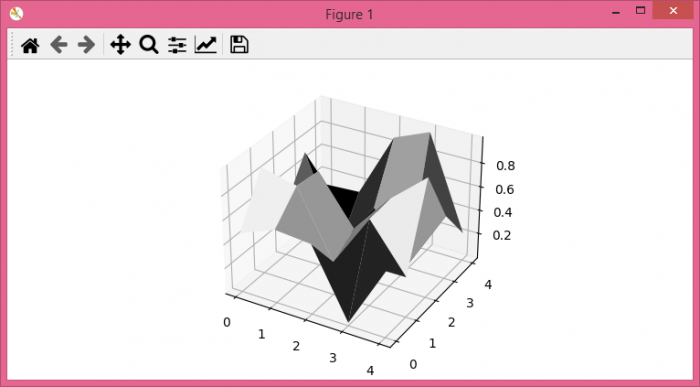# How to create a surface plot from a greyscale image with Matplotlib?

To create a surface plot from a grayscale image with matplotlib, we can take the following steps −

• Set the figure size and adjust the padding between and around the subplots.

• Create random data points using Numpy.

• Get the xx and yy data points from a 2d image data raster.

• Create a new figure or activate an existing figure.

• Get the current axis of the plot and make it 3d projection axes.

• Create a surface plot with cmap='gray'.

• To display the figure, use show() method.

## Example

import numpy as np
import matplotlib.pyplot as plt

plt.rcParams["figure.figsize"] = [7.50, 3.50]
plt.rcParams["figure.autolayout"] = True

data = np.random.rand(5, 5)

xx, yy = np.mgrid[0:data.shape, 0:data.shape]

fig = plt.figure()

ax = fig.gca(projection='3d')
ax.plot_surface(xx, yy, data, rstride=1, cstride=1, linewidth=0, cmap='gray')

plt.show()

## Output